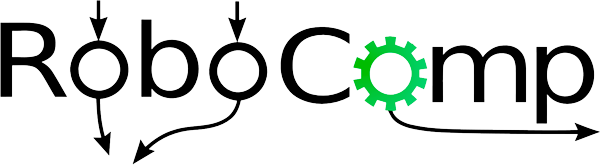# Naive Bayes Predictor API

The Naive Bayes Predictor used in the current project uses a specific encoding to convert planning data to variables of the classifier. We’ve created this API for easy integration of other encodings.

As of now, there are two classes `NaiveBayesClassifier` and `NaiveBayesInputValidator`. The second class does some validation (matrix dimensions, values etc.) of inputs passed to first class.

The first class `NaiveBayesClassifier` class takes minimalistic input:

• Number of times an attribute was observed given a target (attr_freq).
• Number of times a target was observed (target_freq).
• Laplace Constant (Optional) for handling variables that weren’t observed while training.
• File Name (Optional) which when unpickled should return a tuple with value (attr_freq, target_freq).

For getting probability distribution over target variables, one must call `predict()` function of `NaiveBayesClassifier`. Input of the function consists of attribute list and a flag-value [boolean] (Optional) which if true (default and suggested) normalizes the output.

Note: The API assumes that all variables involved must take take binary values. Therefore the attribute value of the variable passed in the matrix, basically corresponds it being to true. It’s absence means the value is false. As said before the flexibility that API provides is in terms of the encoding of training variables.

Lashit Jain